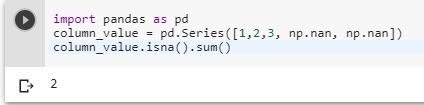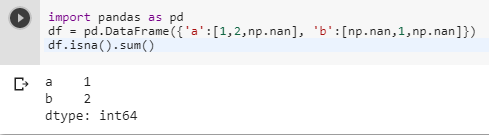# How to count the NaN values in a column in pandas DataFrame

1 view
in Python

I have data, in which I want to find the number of NaN so that if it is less than some threshold, I will drop these columns. I looked but wasn't able to find any function for this. there are value_counts, but it would be slow for me because most of the values are distinct and I want the count of NaN only.

by (107k points)

If you want to count the NaN values in a column in pandas DataFrame you can use the isna() method or it's alias isnull() method the isnull() method is compatible with older pandas versions < 0.21.0 and then sum to count the NaN values. For one column:

import pandas as pd

column_value = pd.Series([1,2,3, np.nan, np.nan])

column_value.isna().sum()If your code has many columns then you can use the following code to count the NaN values this code will return you the name of the column which contains the NaN value as well as the data types:-

import pandas as pd

df = pd.DataFrame({'a':[1,2,np.nan], 'b':[np.nan,1,np.nan]})

df.isna().sum()